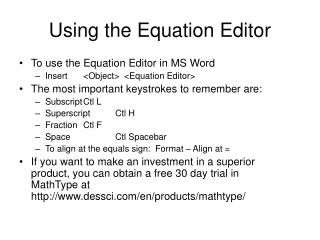DownloadDownload PresentationUsing the Equation Editor

# Using the Equation Editor

Télécharger la présentation## Using the Equation Editor

- - - - - - - - - - - - - - - - - - - - - - - - - - - E N D - - - - - - - - - - - - - - - - - - - - - - - - - - -
##### Presentation Transcript

1. Using the Equation Editor • To use the Equation Editor in MS Word • Insert <Object> <Equation Editor> • The most important keystrokes to remember are: • Subscript Ctl L • Superscript Ctl H • Fraction Ctl F • Space Ctl Spacebar • To align at the equals sign: Format – Align at = • If you want to make an investment in a superior product, you can obtain a free 30 day trial in MathType at http://www.dessci.com/en/products/mathtype/

2. Practice Problem: Chap 9 • You are analyzing two projects. Their cash flows are as follows: 0 1 2 3 4 • Project A: -100 25 40 60 100 • Project B: -100 80 40 20 10 • For each project, calculate: • NPV (cost of capital is 12%) • Payback • Discounted payback • IRR • Profitability Index • Draw the NPV profile for both projects on one graph